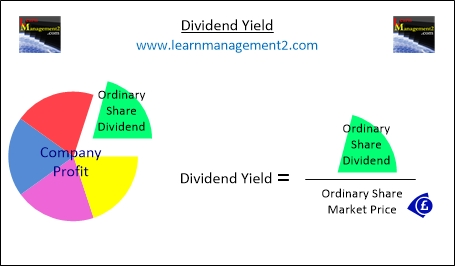# Dividend Yield

Firms don't always distribute all of the profit it makes to shareholders, they will keep money back for future investments and emergency reserves. This means that even if the earnings per share seems to be a reasonable amount, it doesn't’mean that the shareholders have made a good return on their investment. Instead you need to calculate the dividend yield if you would like an indication of how well the return on shares compares to the price of a share.The diagram above shows how to calculate the dividend yield, using: 1)The ordinary share dividend amount and 2) The market price of an ordinary share

### What Is The Dividend Yield?

The dividend yield compares the amount of money ordinary share shareholders receive in the form of dividends, against the market price of an ordinary share. The dividend yield is a useful calculation for shareholders to analyse the return they are receiving from their investments.

### How Does the Dividend Yield Differ From Earnings Per Share/Earnings Ratio?

Dividend yield is different to earnings per share and earnings ratio because it takes dividends (the profit that the business has given to shareholders) into account. Dividend yield compares the market price of the share against the dividend paid out by the business.

### Calculating Dividend Yield

To calculate the dividend yield you will need to find out the value of the ordinary share dividend and the ordinary share market price. Once you have these figures divide the ordinary share dividend by the ordinary share market price and multiply the answer by 100%.

Dividend Yield             Ordinary Share Dividend
=       ____________________        x 100%

Ordinary Share Market Price

### Example Calculation

For example if a firm's share market price is £10 (1000p) and they paid out £0.25 (25p) per share the dividend yield would be calculated as follows:

Dividend Yield             25
=       ____    x 100%  =  0.25%

1000

In our example the dividend yield is 0.25% this means that shareholders received 0.25% of the ordinary share market price back as dividends.

### Conclusion

The dividend yield will change as and when the ordinary share market price changes and when firms issue a dividend. A firm’s share market price depends on the financial strength of the firm, economic conditions and the industry that the firm is based in.